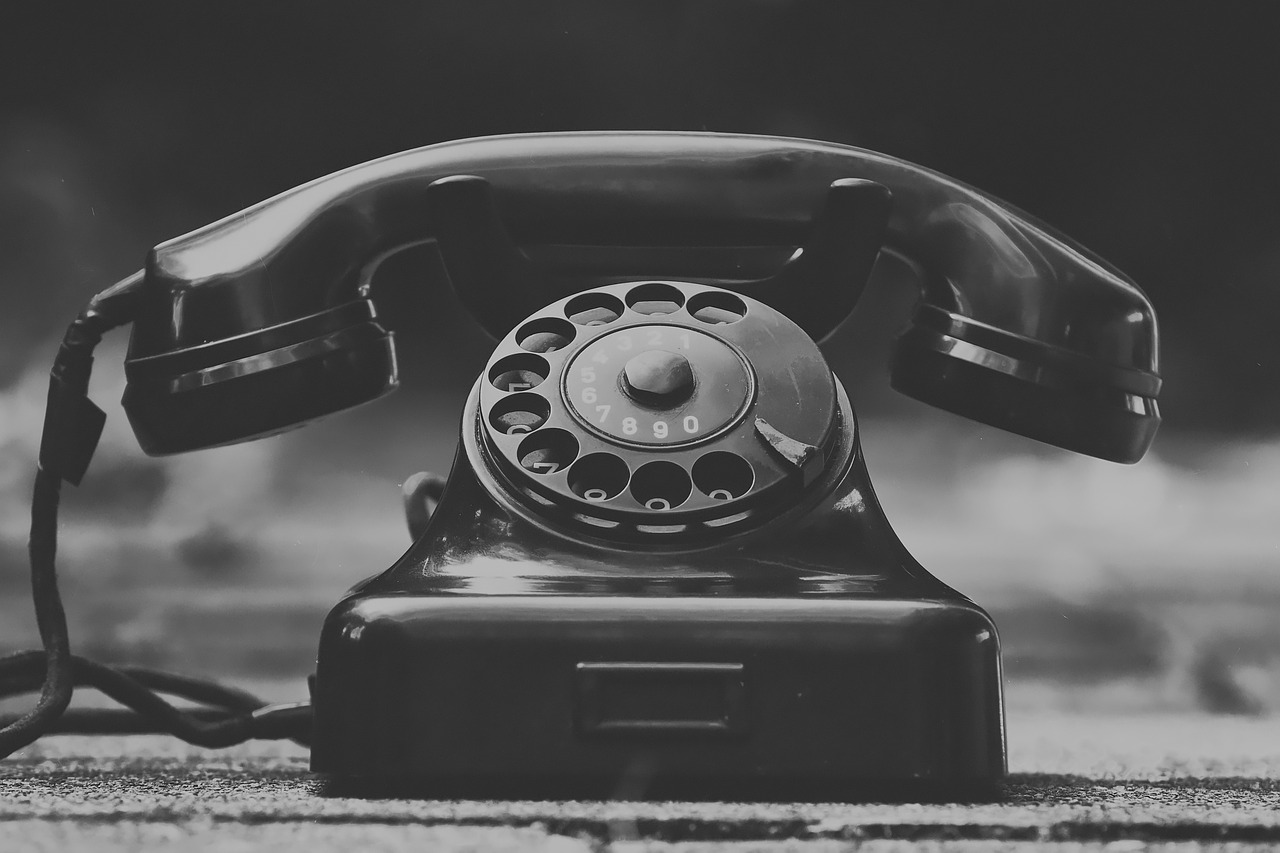## Understanding Decibels & Why OSHA Pays Attention to Your Noise ExposureIn the simplest of metric terms, a decibel is one-tenth of a bel.  But, historically, bel was a unit created to honor Alexander Graham Bell who invented the telephone.  In the early days with telephone wires, they noticed that the signal strength would decay over a long distance.  In order to determine power requirements to connect people for communications, they determined that they could use the ratio of power levels.  As a start, it had to be based on a minimum amount of power required for a person to hear on the telephone.  They found that the signal power level to generate an angular frequency of 5000 radians per second would be that minimum value as determined by an average number of people.  They used this mark as a reference point in the ratio of power levels.  Because of the large variations in values, they simplified the equation on a base-10 log scale and dividing the bel unit by 10.  Thus, creating the measurement of decibel.

Today, this same method is used to measure sound.  Like frequency waves that travel through the telephone wires, pressure waves travel through the air as sound.  This sound pressure is what our ears can detect as loudness, and it has a pressure unit of Pascals (Pa).  As an example, a small sound pressure would be like a whisper while a large sound pressure would be like a jet engine.  This is very important to know as high sound pressures, or loudness, can permanently damage our ears.

With sound pressures, we can determine the Sound Pressure Level (SPL) which is measured in decibels (dB).  Similar to the equation for the telephone power signals above, the SPL also uses a ratio of sound pressures in a base-10 logarithmic scale.  For a minimum reference point, an average human can just start to hear a sound pressure at 0.00002 Pa.  So, the equation for measuring sound levels will use this minimum reference point as shown in Equation 1.

Equation 1:

L = 20 * Log10 (p/pref)

where:

L – Sound Pressure Level, dB

p – Sound pressure, Pa

pref – reference sound pressure, 0.00002 Pa

Why is this important to know the decibels?  OSHA created a chart in 29CFR-1910.95(a) that shows the different noise levels with exposure times.  This chart was created to protect the operators from hearing loss in work environments.  If the noise level exceeds the limit, then the operators will have to wear Personal Protection Equipment (PPE), or suffer hearing damage.  EXAIR offers a Sound Level Meter, model 9104, to measure sound levels in decibels.  It comes calibrated to accurately measure the sound to determine if you have a safe work environment.